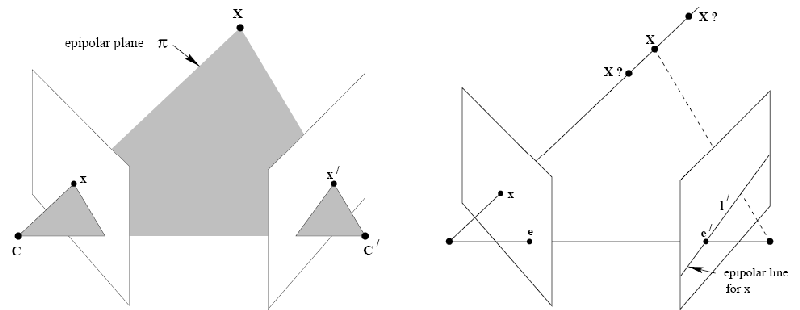# Introduction

It is expected that you know some basics of projective geometry as well as epipolar geometry both of which are essential to understand stereo vision. I will not try to explain the derivations in detail here - you can read about them in detail in the book suggested in the references section.

I will show the closed form solution of the fundamental matrix, given two camera matrices of the stereo setup. Also the rotation and translation matrices between the two cameras should be known for this.

I have also provided a GNU Octave code to quickly calculate the fundamental matrix.

# Deriving the fundamental matrixConsider the above image. $\mathbf{C}$ and $\mathbf{C’}$ are the camera centers of the left and right camera respectively. The corresponding point for $\mathbf{x}$ (left image) is $\mathbf{x’}$ in the right image. $\mathbf{x’}$ is guaranteed to lie on the epipolar line $\mathbf{l’}$. $\mathbf{X}$ is the coordinate of the point in 3D space. $\mathbf{e}$ and $\mathbf{e’}$ are the epipoles.

Note: We are working in homogeneous coordinates here.

We define the fundamental matrix $\mathbf{F}$ as a mapping from a point in an image plane to an epipolar line in the other image.

$\mathbf{l'} = \mathbf{F}\mathbf{x}$

The form of the fundamental matrix in terms of the two camera projection matrices, $\mathbf{P}$, $\mathbf{P’}$, may be derived algebraically. The ray back-projected from $\mathbf{x}$ by $\mathbf{P}$ is obtained by solving $\mathbf{P}$$\mathbf{X}$ = $\mathbf{x}$. The one-parameter family of solutions is of the form given by

$\mathbf{X(\lambda) = \mathbf{P}^+\mathbf{x} + \lambda\mathbf{C}}$

where $\mathbf{P}^+$ is the pseudo-inverse of $\mathbf{P}$, i.e. $\mathbf{PP}^+ = \mathbf{I}$, and $\mathbf{C}$ its null-vector, namely the camera centre, defined by $\mathbf{PC} = \mathbf{0}$. The ray is parametrized by the scalar $\lambda$. In particular two points on the ray are $\mathbf{P}^+\mathbf{x}$ (at $\lambda = 0$), and the first camera centre $\mathbf{C}$ (at $\lambda = \infty$). These two points are imaged by the second camera $\mathbf{P’}$ at $\mathbf{P’P}^+\mathbf{x}$ and $\mathbf{P’C}$ respectively in the second view. The epipolar line is the line joining these two projected points, namely $\mathbf{l’} = (\mathbf{P’C})\times (\mathbf{P’P}^+\mathbf{x})$. The point $\mathbf{P’C}$ is the epipole in the second image, namely the projection of the first camera centre, denoted by $\mathbf{e’}$. Thus, $\mathbf{l’} = [\mathbf{e’}]_{\times}\mathbf{P’P}^+\mathbf{x} = \mathbf{Fx}$

$\mathbf{F} = [\mathbf{e'}]_{\times}\mathbf{P'P}^+$

where $[\mathbf{e’}]_{\times}$ is a cross product matrix.

Now the cameras are calibrated, and let’s assume the world origin is at the left camera.

$\mathbf{P} = \mathbf{K}\left[\begin{array}{c|c} \mathbf{I} & \mathbf{0} \\ \end{array}\right] \quad \quad \mathbf{P'} = \mathbf{K'}\left[\begin{array}{c|c} \mathbf{R} & \mathbf{t} \\ \end{array}\right]$

Then,

$\mathbf{P}^+ = \begin{bmatrix} \mathbf{K}^{-1} \\ \mathbf{0}^\top \\ \end{bmatrix} \quad \quad \mathbf{C} = \begin{bmatrix} \mathbf{0} \\ 1 \end{bmatrix}$

and

$\mathbf{F} = [\mathbf{P'C}]_{\times}\mathbf{P'P}^+ = [\mathbf{K't}]_{\times}\mathbf{K'RK}^{-1} = \mathbf{K'}^{-\top}[\mathbf{t}]_{\times}\mathbf{RK}^{-1} = \mathbf{K'}^{-\top}\mathbf{R}[\mathbf{R}^{\top}\mathbf{t}]_{\times}\mathbf{K}^{-1} = \mathbf{K'}^{-\top}\mathbf{RK}^{\top}[\mathbf{KR}^{\top}\mathbf{t}]_{\times}$

Hence we derived an expression for $\mathbf{F}$ purely in terms of $\mathbf{K}$, $\mathbf{K’}$, $\mathbf{R}$, and $\mathbf{t}$. The fundamental matrix relates two point correspondences in the left and right image. The relation is given as

$\mathbf{x'^{\top}Fx} = 0$

# Octave Code

C is the cross product matrix of A. Note the structure of C. This function returns the $3 \times 3$ fundamental matrix. This can easily be implemented in C++ and OpenCV as well.

Multiple View Geometry in Computer Vision, by Richard Hartley and Andrew Zisserman